Dersleri yüzünden oldukça stresli bir ruh haline sikiş hikayeleri bürünüp özel matematik dersinden önce rahatlayabilmek için amatör pornolar kendisini yatak odasına kapatan genç adam telefonundan porno resimleri açtığı porno filmini keyifle seyir ederek yatağını mobil porno okşar ruh dinlendirici olduğunu iddia ettikleri özel sex resim bir masaj salonunda çalışan genç masör hem sağlık hem de huzur sikiş için gelip masaj yaptıracak olan kadını gördüğünde porn nutku tutulur tüm gün boyu seksi lezbiyenleri sikiş dikizleyerek onları en savunmasız anlarında fotoğraflayan azılı erkek lavaboya geçerek fotoğraflara bakıp koca yarağını keyifle okşamaya başlar

GET THE APP

Hard Decision Based Cooperative Spectrum Sensing over Different Fading Channel in Cognitive Radio | OMICS International
International Journal of Advance Innovations, Thoughts & Ideas

# Hard Decision Based Cooperative Spectrum Sensing over Different Fading Channel in Cognitive Radio

Md. Shamim Hossain1*,Sharif Mohammed Babul Haque2,Md. Ibrahim Abdullah3 and Mohammad Alamgir Hossain4

1Lecturer, Dept. of CSE, Islamic University, Kushtia, Bangladesh.

2Assistant Registrar, Atish Dipankar University of Science & Technology, Dhaka, Bangladesh.

3Associate Professor, Dept. of CSE, Islamic University, Kushtia, Bangladesh.

4Lecturer, Dept. of CSE, Atish Dipankar University of Science & Technology, Dhaka, Bangladesh.

*Corresponding Author:
Md. Shamim Hossain
Lecturer, Dept. of CSE, Islamic University, Kushtia, Bangladesh.
E-mail:
shamimmalitha@yahoo.com

Visit for more related articles at International Journal of Advance Innovations, Thoughts & Ideas

#### Keywords

Cognitive Radio, Hard decision fusion rules, Cooperative spectrum sensing, fading channels, Energy detection

#### Introduction

The rest of this paper is organized as follows. In Section II, the system model is introduced. In Section III, detection and false alarm probabilities of non-fading AWGN and fading channel such as Rayleigh, Ricean and Nakagami are described. Cooperative spectrum sensing over various fading channels is derived in Section IV. The simulation result and discussion are presented in section V. Finally, we draw our conclusions in Section VI.

#### System Model

The local spectrum sensing is to decide between the following two hypotheses,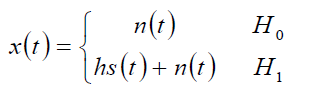(1)

where x(t) is the signal received by secondary user and s(t) is primary user’s transmitted signal, n(t) is the additive white Gaussian noise (AWGN) and h is the amplitude gain of the channel. The energy collected in the frequency domain is denoted by Y which serves as a decision statistic. Following the work of Urkowitz , Y may be shown to have the following distribution,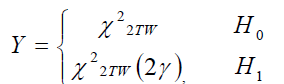(2)

where and denote central and non-central chi-square distributions respectively, each with 2TW degrees of freedom and a non-centrality parameter of 2γ for the latter distribution. For simplicity we assume that time-bandwidth product, TW, is an integer number which we denote by u.

#### Detection and False Alarm Probabilities

In this section, we give the average detection probability over Rayleigh, Nakagami, and Ricean fading channels and in closed form . In communications theory, Nakagami distributions, Rician distributions, and Rayleigh distributions are used to model scattered signals that reach a receiver by multiple paths. Depending on the density of the scatter, the signal will display different fading characteristics. Rayleigh and Nakagami distributions are used to model dense scatters, while Rician distributions model fading with a stronger line-of-sight. Nakagami distributions can be reduced to Rayleigh distributions, but give more control over the extent of the fading.

In non-fading environment the average probability of false alarm, the average probability of detection, and the average probability of missed detection are given, respectively, by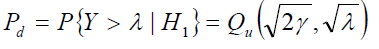(3)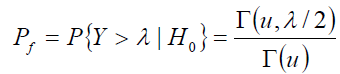(4)

and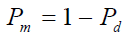(5)

where λ denotes the energy threshold. Γ(.) and Γ(.,.) are complete and incomplete gamma functions respectively  and (.,.) Qu is the generalized Marcum Q-function defined as follows,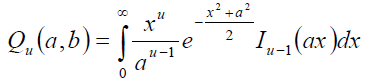where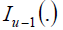is the modified Bessel function of (u−1)th order. If the signal power is unknown, we can first set the false alarm probability Pf to a specific constant. By equation (4), the detection threshold λ can be determined. Then, for the fixed number of samples 2TW the detection probability Pd can be evaluated by substituting the λ in (3). As expected, Pf is independent of γ since under H0 there is no primary signal present. When h is varying due to fading, equation (3) gives the probability of detection as a function of the instantaneous SNR, γ. In this case, the average probability of detection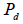may be derived by averaging (3) over fading statistics ,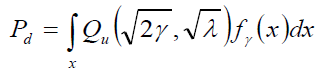(6)

where fγ(x) is the probability distribution function (PDF) of SNR under fading.

When the composite received signal consists of a large number of plane waves, for some types of scattering environments, the received signal has a Rayleigh distribution . If the signal amplitude follows a Rayleigh distribution, then the SNR γ follows an exponential PDF given by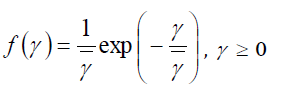(7)

In this case, a closed-form formula for d P may be obtained (after some manipulation) by substituting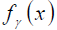in (6),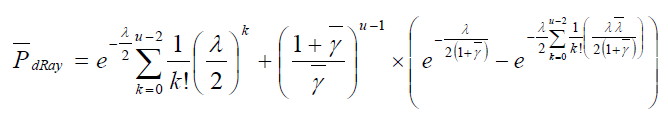(8)

Some types of scattering environments have a specular or LoS (Line of Sight) component. In this case, the amplitude of received signals has a Ricean distribution. If the signal strength follows a Rician distribution, the PDF of γ will be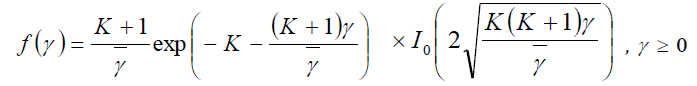(9)

where K is the Rician factor. The average Pd in the case of a Rician channel,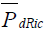is then obtained by averaging (3) over (9) and substituting x for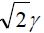The resulting expression can be solved for u = 1 using , Eq. (45)] to yield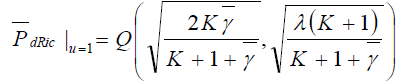(10)

For K = 0, this expression reduces to the Rayleigh expression with u = 1.

Although Rayleigh and Ricean distributions are the most popular distributions to model fading channels, some experimental data does not fit well into neither of these distributions. Thus, a more general fading distribution was developed whose parameters can be adjusted to fit a variety of empirical measurements . This distribution is called the Nakagami fading distribution. The Nakagami distribution was introduced by Nakagami in the early 1940’s to characterize rapid fading in long distance HF channels . It is possible to describe both Rayleigh and Rician fading with the help of a single model using the Nakagami distribution. The Nakagami m-distribution is used in communication systems characterize the statistics of signal transmitted through multipath fading channels.

The Nakagami distribution is often used for the following reasons. First, the Nakagami distribution can model fading conditions that are either more or less severe than Rayleigh fading. When m=1, the Nakagami distribution becomes the Rayleigh distribution, when m=1/2, it becomes a one-sided Gaussian distribution, and when m=∞ the distribution becomes an impulse (no fading). Second, the Rice distribution can be closely approximated by using the following relation between the Rice factor K and the Nakagami shape factor m ;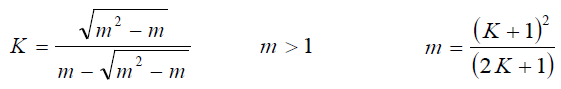Since the Rice distribution contains a Bessel function while the Nakagami distribution does not, the Nakagami distribution often leads to convenient closed form analytical expressions that are otherwise unattainable. Using the alternative representation of Marcum-Q function given in [28, eq. (4.74), pp. 104], (1) can be written as,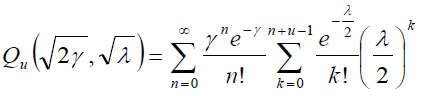(11)

If the signal amplitude follows a Nakagami distribution, then the PDF of γ follows a gamma PDF given by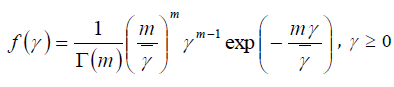(12)

where m is the Nakagami parameter. The average Pd in the case of Nakagami channels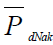can now be obtained by averaging (3) over (12) and then using again the change of variable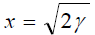yielding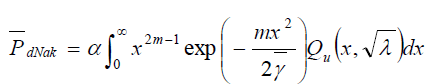(13)

where(14)

In this case, a closed-form formula of Nakagami channels can be given by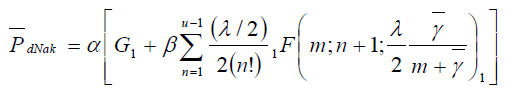(15)

where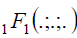is the confluent hypergeometric function .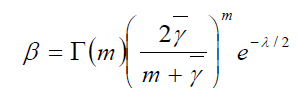(16)

and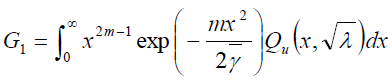(17)

Where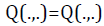is the first-order Marcum Q-function. G1 can be evaluated for inter m with the aid of [25, Eq.(25)]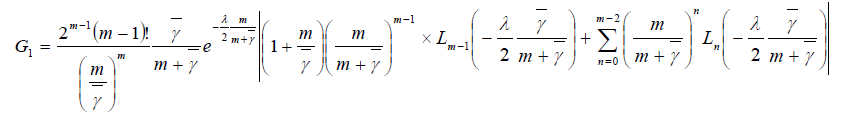(18)

where is the Laguerre polynomial of degree n [25, 8.970].

#### Cooperative Spectrum Sensing over various Fading Channels

In real communication environments, the hidden terminal problem, deep fading and shadowing, etc., would deteriorate the signal detection performance of cognitive users. To address this issue, multiple cognitive radios can be coordinated to perform spectrum sensing. Several recent works have shown that cooperative spectrum sensing can greatly increase the probability of detection in fading channels , .

Let N denote the number of users sensing the PU. Each CR user makes its own decision regarding whether the primary user present or not, and forwards the binary decision (1 or 0) to fusion center (FC) for data fusion. The PU is located far away from all CRs. All the CR users receive the primary signal with same local mean signal power, i.e. all CRs form a cluster with distance between any two CRs negligible compared to the distance from the PU to a CR. For simplicity we have assumed that the noise, fading statistics and average SNR are the same for each CR user. We consider that the channels between CRs and FC are ideal channels (noiseless). Assuming independent decisions, the fusion problem where k out of N CR users are needed for decision can be described by binomial distribution based on Bernoulli trials where each trial represents the decision process of each CR user. With a hard decision counting rule, the fusion center implements an n–outof- M rule that decides on the signal present hypothesis whenever at least k out of the N CR user decisions indicate . Assuming uncorrelated decisions, the probability of detection at the fusion center  is given by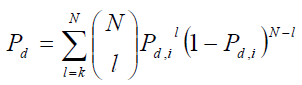(19)

where Pd,i is the probability of detection for each individual CR user as defined by (3) and (6).

Logical AND-Rule

In this rule, if all of the local decisions sent to the decision maker are one, the final decision made by the decision maker is one. The fusion center’s decision is calculated by logic AND of the received hard decision statistics. Cooperative detection performance with this fusion rule can be evaluated by setting k=N in eq. (19).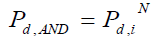(20)

Logical OR-Rule

In this rule, if any one of the local decisions sent to the decision maker is a logical one, the final decision made by the decision maker is one. Cooperative detection performance with this fusion rule can be evaluated by setting k=1 in eq. (19).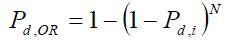(21)

Logical MAJORITY -Rule

In this rule, if half or more of the local decisions sent to the decision maker are the final decision made by the decision maker is one. Cooperative detection performance with this fusion rule can be evaluated by setting k = N/2 in eq. (19).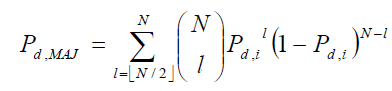(22)

where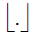represents the floor operator.

#### Experimental Results

All simulation was done on MATLAB version R2011a over three different fading under Rayleigh, Ricean and Nakagami channel and a non-fading channel AWGN. We described the receiver through its complementary ROC curves for different values of probability of false alarm and Cognitive Radio user.

Fig. 2(a), 2(b) and 2(c) show complementary ROC curves of the 10 user’s spectrum sensing in three different fading under Rayleigh, Ricean and Nakagami fading following AND rule, OR rule and MAJORITY rule respectively. Average SNR and u are assumed to be 10 dB and 5 respectively. Rice factor k and Nakagami parameter m are set to be 5 and 3 respectively. A plot for non-fading (pure AWGN) case is also provided for comparison.

Figure 2a: Complementary ROC of AND fusion rule over different fading channels (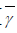=10dB, N=10, u=5, k=5, m=3).

Figure 2b: Complementary ROC of OR fusion rule over different fading channels(=10dB, N=10, u=5, k=5, m=3).

Figure 2c: Complementary ROC of MAJORITY fusion rule over different fading channels(=10dB, N=10, u=5, k=5, m=3).

Comparing the AWGN curve with those corresponding to fading, we observe that spectrum sensing is harder in presence of Rayleigh and Nakagami fading. In Ricean channel, because of the LoS signal, the sensing performance is better than in other channels. We observe that the OR rule has the better performance than AND and MAJORITY rule in various channels.

Fig. 3(a), 3(b), 3(c) and 3(d) show complementary ROC of hard decision fusion rule(AND-rule, OR-rule and MAJORITY-rule) of 10 user’s spectrum sensing in non-fading AWGN and three different fading under Rayleigh, Ricean and Nakagami fading respectively. A plot for single user’s spectrum sensing is also provided for comparison. As before γ = 10 dB, u =5, k = 5, m = 3.

Figure 3a: Complementary ROC of hard fusion rule over non-fading AWGN channel for 10 user(=10dB, u=5).

Figure 3b: Complementary ROC of hard fusion rule over Ricean fading channel for 10 user(=10dB, u=5, k=5).

Figure 3c: Complementary ROC of hard fusion rule over Rayleigh fading channel for 10 user(=10dB, u=5).

Figure 3d: Complementary ROC of hard fusion rule over Nakagami fading channel for 10 user(=10dB, u=5, m=5).

Simulation result shows that probability of missed detection of AND rule is larger than missed detection of single user over various channels. It also shows that OR rule has the better performance than AND and MAJORITY rule. Comparing the AWGN curve with those corresponding to fading, we observe that spectrum sensing is harder in presence of Rayleigh and Nakagami fading.

Fig. 4(a), 4(b), 4(c) and 4(d) show the complementary ROC of hard decision fusion OR rule for different number of cooperative users of cooperative spectrum sensing over non-fading AWGN channel and three different fading such as Ricean, Rayleigh and Nakagami fading channel respectively. A plot for single user’s spectrum sensing is also provided for comparison. As before γ = 10 dB, u =5, k = 5, m = 3.

Figure 4a: Complementary ROC over non-fading AWGN channel for different number of users(=10dB, u=5).

Figure 4b: Complementary ROC over Ricean fading channel for different number of CR users(=10dB, u=5, k=5).

Figure 4c: Complementary ROC over Rayleigh fading channel for different number of CR users(=10dB, u=5).

Figure 4d: Complementary ROC over Nakagami fading channel for different number of CR users(=10dB, u=5).

Simulation result shows that cooperative sensing performance is getting better with increasing CR user as for larger CR user, with high probability there will be a user with a preferable channel to find the presence of PU.

#### Conclusion & Future Work

We have studied hard decision based cooperative spectrum sensing over different fading channel in cognitive radio. Performance of cooperative spectrum sensing over Rayleigh, Ricean and Nakagami fading are presented and compared. It has been found that probability of missed detection is decreased by using different hard decision fusion rules. We observe that the OR rule has the better performance than AND and MAJORITY rule in various channels. We also observe that spectrum sensing is harder in presence of Rayleigh and Nakagami fading and performance of energy detection degrades more in Nakagami channels than Rayleigh and Ricean channels. In Ricean channel, because of the LoS signal, the sensing performance is better than in other channels. Furthermore, spectrum sensing in Ricean fading has better results than others.

--

### Article Usage

• Total views: 14638
• [From(publication date):
May-2013 - Dec 02, 2023]
• Breakdown by view type
• HTML page views : 10155Can't read the image? click here to refresh